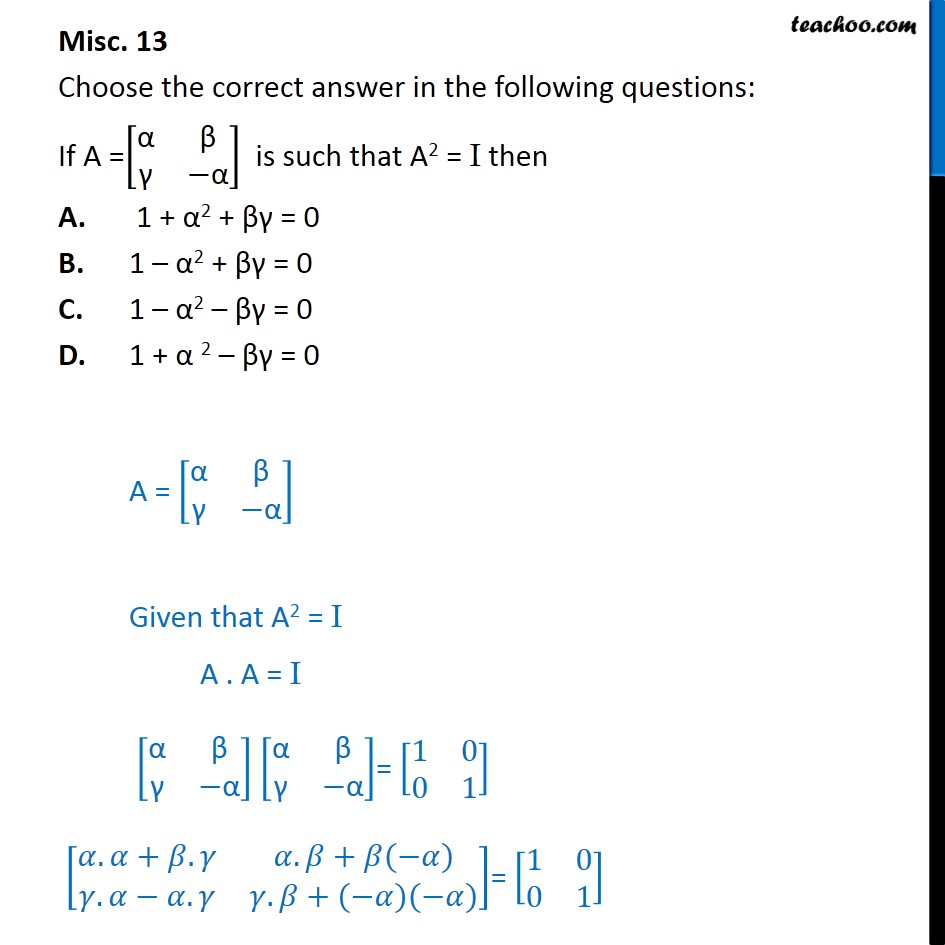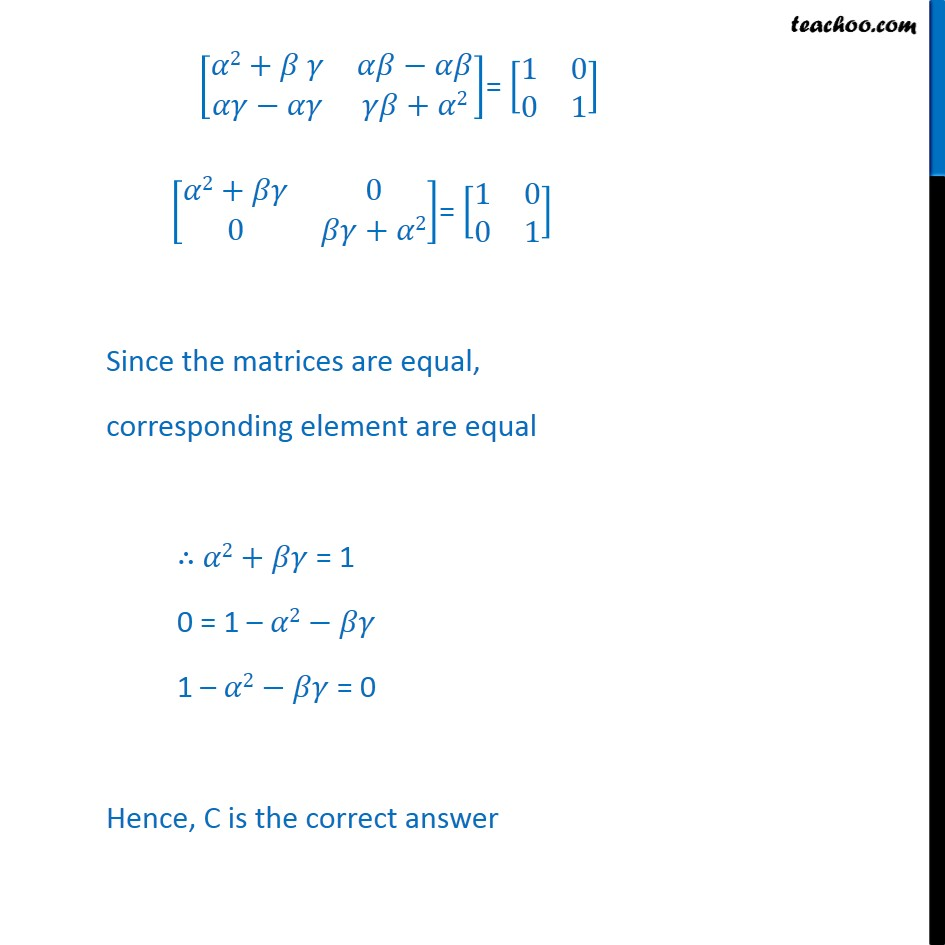1. Class 12
2. Important Question for exams Class 12
3. Chapter 3 Class 12 Matrices

Transcript

Misc. 13 Choose the correct answer in the following questions: If A =[■8("α" &"β" @"γ" &−"α" )] is such that A2 = I then A. 1 + α2 + βγ = 0 B. 1 – α2 + βγ = 0 C. 1 – α2 – βγ = 0 D. 1 + α 2 – βγ = 0 A = [■8("α" &"β" @"γ" &−"α" )] Given that A2 = I A . A = I [■8("α" &"β" @"γ" &−"α" )] [■8("α" &"β" @"γ" &−"α" )]= [■8(1&0@0&1)] [■8(𝛼. 𝛼+𝛽. 𝛾&𝛼. 𝛽+𝛽(−𝛼)@𝛾. 𝛼−𝛼. 𝛾&𝛾. 𝛽+(−𝛼)(−𝛼))]= [■8(1&0@0&1)] [■8(𝛼2+𝛽 𝛾&𝛼𝛽−𝛼𝛽@𝛼𝛾−𝛼𝛾&𝛾𝛽+𝛼2)]= [■8(1&0@0&1)] [■8(𝛼2+𝛽𝛾&0@0&𝛽𝛾+𝛼2)]= [■8(1&0@0&1)] Since the matrices are equal, corresponding element are equal ∴ 𝛼2+𝛽𝛾 = 1 0 = 1 – 𝛼2−𝛽𝛾 1 – 𝛼2−𝛽𝛾 = 0 Hence, C is the correct answer

Chapter 3 Class 12 Matrices

Class 12
Important Question for exams Class 12